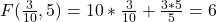Question

Given that xy =3/2 and both x and y are nonnegative real numbers, find the minimum value of 10x + 3y/5. Please show steps. Thank you!​

1.First of all, x and y cannot be zero because of the condition xy = 3/2. Hence x and y can only be positive.

Now let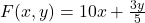Since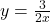, ==> F(x,y) becomes, after substitution,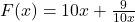==> F'(x) =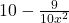Setting this to 0 to find the critical point, we get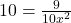or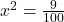==> x = 3/10. (Chose the positive because of the hypothesis.)

==> y = 5.

Now the 2nd derivative is F” =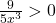hen x = 3/10.

Hence an (absolute) minimum exists at (3/10,5), with value